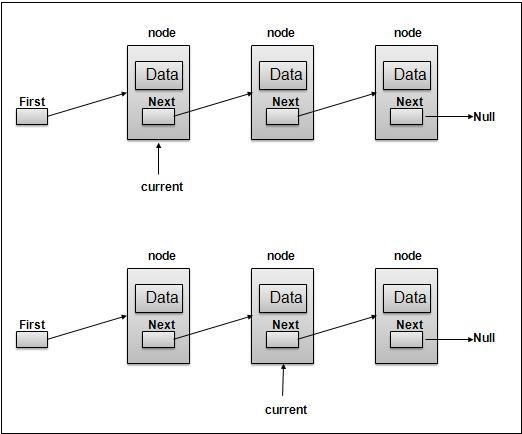# Creating a linked list using Javascript

Let's start by defining a simple class with a constructor that initializes the head to null. We'll also define another structure on the prototype of the LinkedList class that'll represent each node in the linked list.

## Example

class LinkedList {
constructor() {
this.length = 0;
}
}
constructor(data) {
this.data = data; this.next = null;
}
}

Let's also create a display function that'll help us see how our list looks like. This function works as follows.

• It starts from the head.
• It iterates over the list using currElem = currElem.next till currElem doesn't become null, ie, we've not reached the end.
• It prints data for each of the iterations.

Here is an illustration for the same −Now let's have a look at how we'll implement this −

## Example

display() {
}